### Iterative Methods: Gauss-Seidel Method

### The Method

The Gauss-Seidel method offers a slight modification to the Jacobi method which can cause it to converge faster. In the Gauss-Seidel method, the system is solved using forward substitution so that each component uses the most recent value obtained for the previous component. This is different from the Jacobi method where all the components in an iteration are calculated based on the previous iteration. First notice that a linear system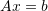of sizecan be written as: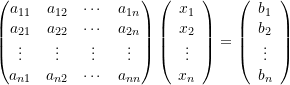The left hand side can be decomposed as follows: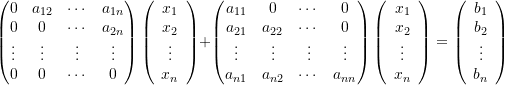Effectively, we have separated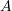into two additive matrices: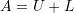where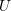is an upper triangular matrix with zero diagonal components, while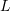is a lower triangular matrix with its diagonal components equal to those of the diagonal components of. If we start with nonzero diagonal components for, thencan be used to solve the system using forward substitution: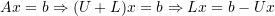the same stopping criterion as in the Jacobi method can be used for the Gauss-Seidel method.

#### Example

Consider the systemdefined as: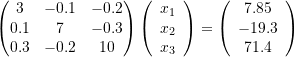The Gauss-Seidel method with a stopping criterion of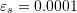will be used. First the system is rearranged to the form: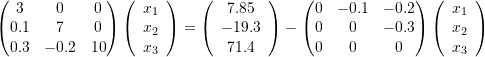Then, the initial guesses for the components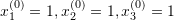are used to calculate the new estimates using forward substitution. Notice that in forward substitution, the value of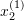uses the value for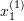and that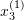uses the values ofand: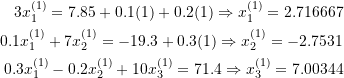The relative approximate error in this case is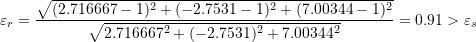For iteration 2: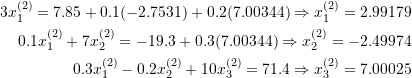The relative approximate error in this case is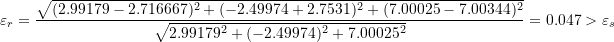For iteration 3: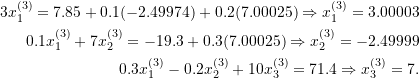The relative approximate error in this case is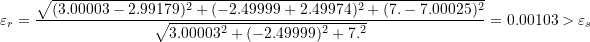And for iteration 4: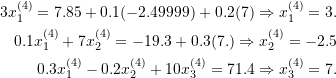The relative approximate error in this case is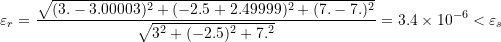Therefore, the system converges after iteration 4 similar to the Jacobi method. It is not obvious in this example, but the Gauss-Seidel method can converge faster than the Jacobi method.

### Programming The Method

Using forward substitution in the equation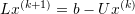, then, the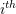component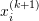in iteration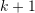can be computed as follows:

(1)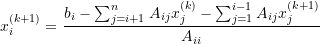The following code utilizes the procedure “GS” that takes a matrix, a vector, and a vectorof some guesses to return the new guess for the components of the vector.

View Mathematica Code
GS[A_, b_, x_] :=
(
n = Length[A];
xnew = Table[0, {i, 1, n}];
Do[xnew[[i]] = (b[[i]] - Sum[A[[i, j]] x[[j]], {j, i + 1, n}] - Sum[A[[i, j]] xnew[[j]], {j, 1, i - 1}])/A[[i, i]], {i, 1, n}];
xnew
)
A = {{3, -0.1, -0.2}, {0.1, 7, -0.3}, {0.3, -0.2, 10}}
b = {7.85, -19.3, 71.4}
x = {{1, 1, 1.}};
MaxIter = 100;
ErrorTable = {1};
eps = 0.001;
i = 2;

While[And[i <= MaxIter, Abs[ErrorTable[[i - 1]]] > eps],
xi = GS[A, b, x[[i - 1]]]; x = Append[x, xi];
ei = Norm[x[[i]] - x[[i - 1]]]/Norm[x[[i]]];
ErrorTable = Append[ErrorTable, ei]; i++]
x // MatrixForm
ErrorTable // MatrixForm

View Python Code
import numpy as np
def GS(A, b, x):
A = np.array(A)
n = len(A)
xnew = np.zeros(n)
for i in range(n):
xnew[i] = (b[i] - sum([A[i, j]*x[j] for j in range(i+1, n)]) - sum([A[i, j]*xnew[j] for j in range(i)]))/A[i, i]
return xnew

A = [[3, -0.1, -0.2], [0.1, 7, -0.3], [0.3, -0.2, 10]]
b = [7.85, -19.3, 71.4]
x = [[1, 1, 1.]]
MaxIter = 100
ErrorTable = 
eps = 0.001
i = 1

while i <= MaxIter and abs(ErrorTable[i - 1]) > eps:
xi = GS(A, b, x[i - 1])
x.append(xi)
ei = np.linalg.norm(x[i] - x[i - 1])/np.linalg.norm(x[i])
ErrorTable.append(ei)
i+=1
print("x:",np.array(x))
print("ErrorTable:",np.vstack(ErrorTable))


The example MATLAB code below is written to emphasize the approach and explicitly works withand. However, the algorithm can be programmed more compactly, for example using the forward substitution form presented in Equation 1.

### Lecture Video

The following video covers the Gauss-Seidel Method